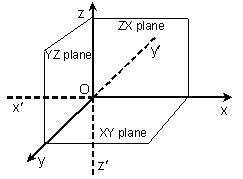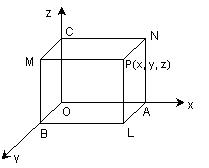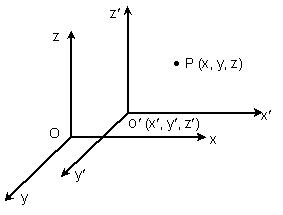Click to Chat

1800-1023-196

+91-120-4616500

CART 0

• 0

MY CART (5)

Use Coupon: CART20 and get 20% off on all online Study Material

ITEM
DETAILS
MRP
DISCOUNT
FINAL PRICE
Total Price: Rs.

There are no items in this cart.
Continue Shopping```Rectangular Coordinate System in Space

Table of Content

Coordinates of a Point in Space

Shifting the Origin

Some Key Points to Remember

Straight Line in Space

Related ResourcesThe coordinate system clearly defines the position of a vector. When we talk of the rectangular coordinates in space, we refer to the three dimensional space we live in. In order to demonstrate the position of a vector first a point is selected as the origin and generally represented by the point ‘O’. The distance of any vector is now measured form this standard point.

Let ‘O’ be any point in space and X’OX, Y’OY and Z’OZ be three lines perpendicular to each other. As shown in the figure below, ‘O’ is called as origin and these three lines denote the coordinate axis. Similarly, the planes XY, YZ and ZX are called the coordinate planes.Coordinates of a Point in Space

Consider a point P in space. The position of the point P is given by the triad (x, y, z) where x, y, z denote the perpendicular distance from YZ-plane, ZX-plane and XY-plane respectively. If the vectors i, j, k  are assumed to be the unit vectors along OX, OY, OZ respectively, then position vector of point P is xi + yj + zk or simply (x, y, z).

We list down certain points which are quite obvious as well as helpful while dealing with a specific coordinate system.

On the x-axis, the y and the z coordinate of a point are always zero. Mathematically, we can write it as x-axis = {( x, y, z) | y = z = 0}

Likewise various other results are as follows:

On y-axis = {(x, y, z) | x = z = 0}

On z-axis = {(x, y, z) | x = y = 0}

On xy plane = {(x, y, z) | z = 0}

On yz plane = {(x, y, z) | x = 0}

On zx plane = {(x, y, z) | y = 0}

Now, if ‘O’ is the origin and P is any point with coordinates (x, y, z) then the distance vector OP by the distance formula is given by OP = √x2 + y2 + z2.Shifting the Origin

At times, certain questions require the shifting of origin without the change in the axis as it simplifies a problem. Such a shift in the origin to another point without the change in the direction of coordinate axis is called as the translation of axis.

We now discuss what exactly happens when the origin is shifted from ‘O’ to another point say O’(x', y', z') without changing the direction of axes.

Let the new coordinate frame be O'X'Y'Z'. Let P (x, y, z) be a point with respect to the coordinate frame OXYZ. Then, coordinate of point P w.r.t. new coordinate frame O'X'Y'Z' is (x1, y1, z1), where

x1 = x – x',

y1 = y – y',

z1 = z – z’

Illustration:

If the origin is shifted to (1, 2, –3) without changing the direction of the axis then find the new coordinates of the point (0, 4, 5) with respect to the new frame.

Solution:

As discussed above, we first assume that the original coordinates of the point were (x, y, z) and after the origin is shifted, the new coordinates of the origin are (x1, y1, z1). Then we have to find the new coordinates of the point say (x', y', z'). Then,x' = x – x1,  where (x1, y1, z1)  is the shifted origin

y' = y – y1

z' = z – z1

x' = 0 – 1 = –1

y' = 4 – 2 = 2

z' = 5 + 3 = 8

∴ The coordinates of the point w.r.t. to new coordinate frame are (-1, 2, 8).

Some Key Points to Remember

Distance between the points P(x1, y1, z1) and Q (x2, y2, z2) is

√(x1 – x2)2 + (y1 – y2)2 + (z1 – z2)2

The point dividing the line joining P(x1, y1, z1) and Q(x2, y2, z2) in m: n ratio is

(mx2 – nx1)/(m + n),

(my2 – ny1)/(m + n),

(mz2 – nz1)/(m + n) where m + n ≠ 0.

The coordinates of centroid of a triangle having vertices A (x1, y1, z1), B (x2, y2, z2) and C (x3, y3, z3) is

G ((x1 + x2 + x3) / 3, (y1 + y2 + y3) / 3, (z1 + z2 + z3)/ 3).

Straight Line in Space

Equation of a line through A(x1, y1, z1) and having direction cosines l, m and n are

(x- x1)/ l = (y - y1)/ m = (z- z1)/ n

And the lines through (x1, y1, z1) and (x2, y2, z2)

(x - x1)/ (x2 - x1) = (y - y1)/ (y2 - y1) = (z - x1)/ (z2 - z1)

Illustration:

Find the coordinates of the point which divides the line joining points (2, 3, 4) and (3, –4, 7) in ratio 3: 5.

Solution:

Let the coordinates of the required point be (x, y, z), then

x = 2(3) + 3(5) / 3 + 5 = 21/8

y = 3(3) – 4(5) / 3 + 5 = – 11/8

z = 4(3) + 7(5) / 3 + 5 = 47/8

Hence the required point is (21/8, –11/8, 47/8).

Illustration:

Prove that the three points A (3, –2, 4), B (1, 1, 1) and C (–1, 4, –2) are collinear.

Solution:

The general coordinates of a point R which divides the line joining A (3, –2, 4) and B (1, 1, 1) in the ratio μ : 1 are

(μ + 3) / (μ + 1), (μ – 2) / (μ + 1), (μ + 4) / μ + 1)       ……(1)

If C (–1, 4, –2) lies on the line AB, then for some value of m the coordinates of μth point R will be the same as those of C.

Let x-coordinate of point R = x - coordinate of point C.

Then, μ + 3 / μ + 1 = –1 => μ = –2

Putting μ = –2 in (1) the coordinates of R are (–1, 4, –2) which are also the coordinates of C.

Hence the points A, B, C are collinear.

Illustration:

A plane passes through (1,-2, 1) and is perpendicular to two planes 2x-2y+z = 0 and x-y+2z = 4, then the distance of the plane from the point (1, 2, 2) is …? (2006)

Solution:

Let the equation of plane be

a(x-1) + b(y+2) + c(z-1) = 0.

This plane is perpendicular to 2x - 2y + z = 0 and x – y + 2z = 4

This gives 2a - 2b + c = 0 and a – b + 2c = 0

Hence, a/-3 = b/-3 = c/0

This implies a/1 = b/1 = c/0

So the equation of the plane is x – 1 + y + 2 = 0 or x + y + 1 = 0

Hence, its distance from the point (1, 2, 2) is |1+2+1| /√2 =

Illustration:

T is a parallelepiped in which A, B, C and D are vertices of one face and the face just above it has corresponding vertices A’, B’, C’, D’. T is now compressed to S with face ABCD remaining same and A’, B’, C’, D’ shifted to A”, B”, C”, D” in S. The volume of parallelepiped S is reduced to 90% of T. Prove that locus of A” is a plane. (2004)

Solution:

Let the equation of the plane ABCD be ax + by + cz + d = 0, the coordinates of the point A” be (α, β, γ) and the height of the parallelopiped ABCD be h.

Then |(aα +b β +c γ + d)|/√(a2 + b2 + c2) = 90%h

⇒ aα +b β +c γ + d = ± 0.9h √(a2 + b2 + c2)

Therefore, locus is ax + by + cz + d = ± 0.9h √(a2 + b2 + c2)

Hence, the locus of A” is a plane parallel to the plane ABCD.

askIITians is an online portal where students can ask their queries on various topics like shifting of origin in coordinate geometry or the rectangular coordinate system.

Related Resources

You may refer the Detailed Syllabus of IIT JEE Mathematics.

Look into the Sample Papers of Previous Years to get a hint of the kind of questions asked in the exam.

The most Useful Books of Mathematics  are just a click away.

To read more, Buy study materials of 3D Geometry comprising study notes, revision notes, video lectures, previous year solved questions etc. Also browse for more study materials on Mathematics here.
```### Course Features

• 731 Video Lectures
• Revision Notes
• Previous Year Papers
• Mind Map
• Study Planner
• NCERT Solutions
• Discussion Forum
• Test paper with Video Solution